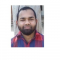## How to create a colored box for base R plot?Updated on 22-Nov-2021 07:29:51
To create a colored box for base R plot, we can use box function with col argument where we can pass the color other than black because black is the default color.We first need to create the plot using plot function and then box function will be used as shown in the below given example.ExampleTo create a colored box for base R plot, use the following code −plot(1:5) box(lwd=5, col="blue")OutputIf you execute the above given code, it generates the following output −To create a colored box for base R plot, use the following code −plot(1:5) box(lwd=10, col="red")OutputIf you execute the ... Read More

## How to create a matrix in R by filling the data with predefined values in loop?Updated on 22-Nov-2021 07:25:17
If we know the total number of rows we want in our matrix and the number of columns then we can use matrix function to create a matrix by filling the data with predefined values. These values must be equal to the multiplication of number of rows and columns.Check out the below given examples to understand how it works.Example 1Following snippet creates a matrix in R by filling the data with predefined values in loop −n=20 k=2 data=rpois(n*k, 5) M1=matrix(data, nrow=n, ncol=k) M1If you execute the above given snippet, it generates the following output −     [, 1] [, 2] ... Read More

## How to create ACF plot in R?Updated on 22-Nov-2021 07:22:14
The autocorrelation plot or ACF plot is a display of serial correlation in data that changes over time. The ACF plot can be easily created by using acf function.For example, if we have a vector called V then we can create its autocorrelation plot by using the command given below −acf(V)Check out the below examples to understand how it can be done.Example 1To create ACF plot in R, use the code given below −x

## How to convert character column of a matrix into numeric in R?Updated on 22-Nov-2021 07:19:54
If we have a matrix that contains character columns and we want to convert a single column to numeric then we first need to convert the matrix into a data frame using as.data.frame function after that as.numeric function can be used to change the particular column to numeric type as shown in the below examples.Example 1Following snippet creates a matrix −M1

## How to find the average of a particular column in R data frame?Updated on 22-Nov-2021 07:12:53
To find the average of a particular column in R data frame, we can take the help of delta ($) operator.For example, if we have a data frame called df that contains a column x then we can find the average of column x by using the command given below −mean(df$x)Example 1Following snippet creates a sample data frame −x1

## How to find the mean of all matrices stored in an R list?Updated on 22-Nov-2021 07:08:25
To find the mean of all matrices stored in an R list, we can use sapply function along with mean function. For example, if we have a list called LIST that contains some matrices then the mean of each matrix can be found by using the command given below −sapply(LIST,mean)Check out the below given example to understand how it works.ExampleFollowing snippet creates a list of matrices −M1

## How to update single value in an R data frame?Updated on 22-Nov-2021 07:04:37
To update single value in an R data frame, we can use row and column indices with single square brackets.For example, if we have a data frame called df that contains two columns and ten rows and if we want to change the fifth value in second column to 10 then we can use the command given below −df[5,2]

## How to find the coordinate of a value in an R matrix?Updated on 22-Nov-2021 07:00:27
The coordinate of a value in an R matrix is the row and column intersection that is the row and column index for that particular value. This can be found by using which function.For example, if we have a matrix called M that contains value starting from 1 to 20 then we can find the coordinate of value 5 by using the command given below − which(M==5,arr.ind=TRUE)ExampleFollowing snippet creates a matrix −M1

## How to fill missing values after merging the data frames with another value than NA in R?Updated on 16-Nov-2021 07:12:20
To fill missing values after merging the data frames with another value than NA in R, we can follow the below steps −First of all, create two data frames.Then, merge the data frames by a common column between the two.After that, replace the NAs with another value.ExampleCreate the first data frameLet’s create a data frame as shown below −ID

## How to use top_n function from dplyr to extract rows based on one column ordered in descending order in R?Updated on 16-Nov-2021 06:53:21
To use top_n function from dplyr to extract rows based on one column ordered indescending order in R, we can follow the below steps −First of all, create a data frame.Then, use top_n function dplyr package along with arrange and desc function to extract rows based on one column ordered in descending order.ExampleCreate the data frameLet’s create a data frame as shown below −x
Previous 1 ... 3 4 5 6 7 ... 202 Next